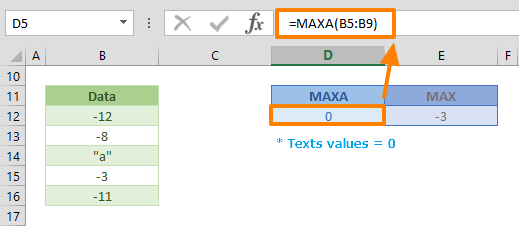The MAXA Excel function returns the maximum value in an array or range of cells. This function works in a similar way as the MAX function. While the MAXA includes logical values in the results, the MAX function ignores them. In this guide, we’re going to show you how to use the MAXA Excel function and also go over some tips and error handling methods.

## Supported versions

• All Excel versions

## MAXA Excel Function Syntax

MAXA(value1,[value2],...)

## Arguments

 value1 The first value or values you want to find the maximum within. You can enter static a value like 4, a cell reference like B6, or a cell range like B2:B8. [value2] Optional. The other value, or values up to 254 arguments.

## MAXA Excel Function Examples

=MAXA(B5:B9)
formula evaluates the values of cells in the reference range. Any valid range can be used with the MAXA function. Our example returns 1, because it evaluates TRUE and FALSE values as 1 and 0 respectively. Thus, the function returns 1, because it’s the largest value in the dataset.This function considers any string value in a data set as 0. As a result, if your range includes a string with negative numbers, the formula in this example returns 0.## Tips

• The MAXA function also evaluates any logical and string values. However; please keep in mind that date or time values are considered to be serial numbers in Excel, not strings.
• TRUE logical value = 1
• FALSE logical value = 0
• String values = 0
• We recommend using the MAX function to ignore anything except for numbers.
• Use the MAXIFS function to find the maximum number that meets specified conditions.
• The opposite of this function is the MINA function, Which returns the minimum number in an array.